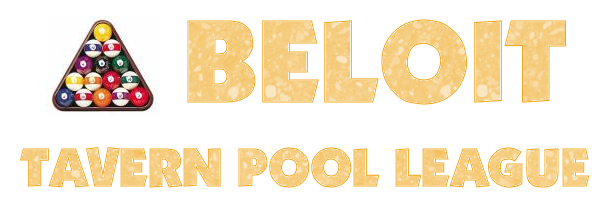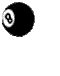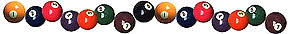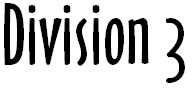SCHEDULE# Wednesday 3'sx

Wednesday 3's Pool

 DIVISION Wolf 1 DOMINICKS 5 TOWN CLUB 2 TURTLE TAP 6 THE RUSTY WHEEL 2 3 CLANCY'S 1 7 PALPABLES 2 4 CASEY'S Bell-ray 8 Bye DIVISION BEAR 1 road dawg pub 5 alarm box 2 the rusty wheel 1 6 the club 3 palpables 1 7 clancy's 2 4 bye 8 jj's 1 October 4, 2023 8 at 1 7 at 2 6 at 3 5 at 4 2 October 11, 2023 8 at 4 3 at 5 2 at 6 1 at 7 3 October 18, 2023 7 at 8 6 at 1 5 at 2 4 at 3 4 October 25, 2023 8 at 3 2 at 4 1 at 5 7 at 6 5 November 1, 2023 6 at 8 5 at 7 4 at 1 3 at 2 6 November 8, 2023 8 at 2 1 at 3 7 at 4 6 at 5 7 November 15, 2023 5 at 8 4 at 6 3 at 7 2 at 1 OFF FOR THANKSGIVING 8 November 29, 2023 1 at 8 2 at 7 3 at 6 4 at 5 9 December 6, 2023 4 at 8 5 at 3 6 at 2 7 at 1 10 December 13, 2023 8 at 7 1 at 6 2 at 5 3 at 4 11 December 20, 2023 3 at 8 4 at 2 5 at 1 6 at 7 OFF FOR CHRISTMAS & NEW YEARS 12 January 3, 2024 8 at 6 7 at 5 1 at 4 2 at 3 13 January 10, 2024 2 at 8 3 at 1 4 at 7 5 at 6 14 January 17, 2024 8 at 5 6 at 4 7 at 3 1 at 2